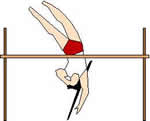Logic puzzles, riddles, math puzzles and brainteasers - pzzls.com

Vandaag is het 26 March 2023

Sort - Most difficult on top
Page: << 1 2 3 4 5 >>

# Einstein as a speed devil - science puzzle

Difficulty:Rating: 3.1/5.0

 How fast should you drive towards a red traffic light to see it appear as green?# Pole vaulting - science puzzle

Difficulty:Rating: 2.9/5.0

 What is the maximal height a pole vaulter could theoretically jump? Would it ever be possible that a pole vaulter passes the height of 10 meter?# Voltage voodoo - science puzzle

Difficulty:Rating: 3.1/5.0

 In the figure, an electrical circuit is drawn. In the middle of the circuit a infinitely long metal bar is placed indicated with the grey circle. In this bar, an oscillating magnetic field is induced by a coil which is attached somewhere to the bar. Two resistors are located in the circuit with values R1 and R2. The alternating voltages V1 and V2 are being measured with two perfect alternating voltages meters. What is the relation between the two voltages V1 and V2? a) V1 : V2 = R2 : R1 b) V1 : V2 = 1 : 1 c) V1 : V2 = R1 : R2 d) V1 = V2 = 0 e) something different.